Note: The other languages of the website are Google-translated. Back to English

## How to calculate Easter date in Excel?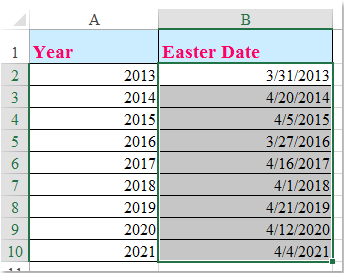As we all known, Easter holiday is always the first Sunday which after the first ecclesiastical full moon that occurs on or after March 21, so the Easter date of each year may be different date as following screenshot shown. To get the specific Easter date of a given year, this article may do you a favor.

Calculate Easter date of given year with formula

Calculate Easter date of given year with User Defined Function

####Calculate Easter date of given year with formula

To get the Easter date of given year, the following formula can help you, please do as follows:

1. Enter this formula: =FLOOR("5/"&DAY(MINUTE(A2/38)/2+56)&"/"&A2,7)-34 into a blank cell beside your given year, B2, for example, see screenshot: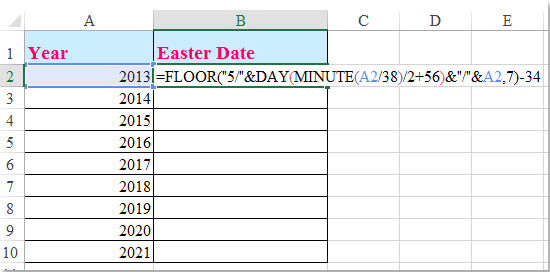2. Then drag the fill handle down to the cells that you want to calculate the Easter date of the given date, and five-digit numbers are displayed in the cells, see screenshot: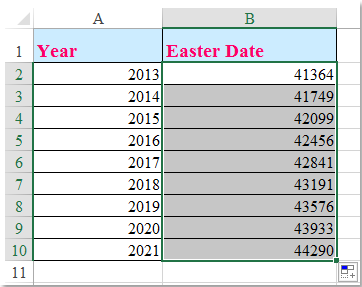3. And then you should format he numbers as date format:

(1.) Select the 5-digit numbers and right click to choose Format Cells from the context menu;

(2.) In the popped out Format Cells dialog box, click Number tab, and select Date from the Category pane, then choose one date format you like from the right list box, see screenshot: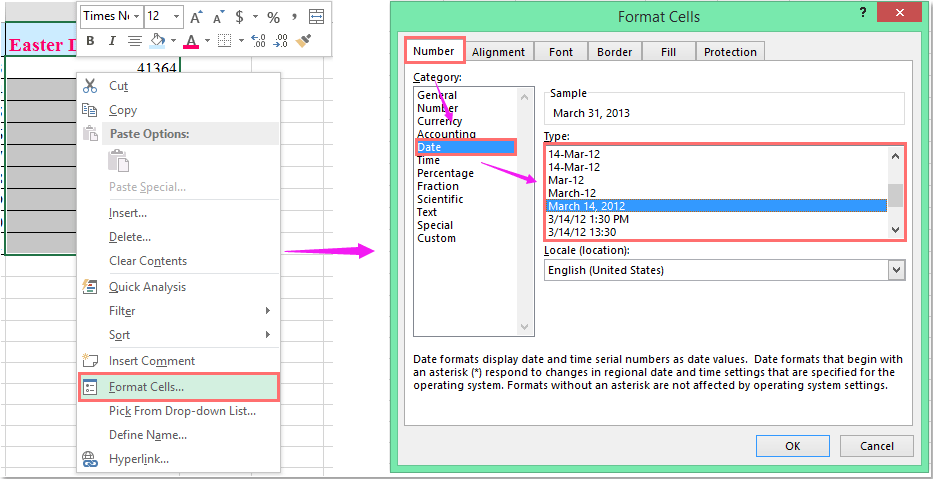4. And then, the numbers have been formatted as the date format as you need, and you can get the Easter date of the given year, see screenshot: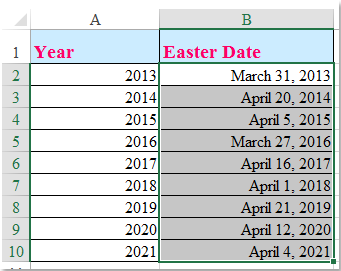Tips: To format the numbers to your normal used date format, you can also use the Apply Date Formatting utility of Kutools for Excel as following screenshot shown: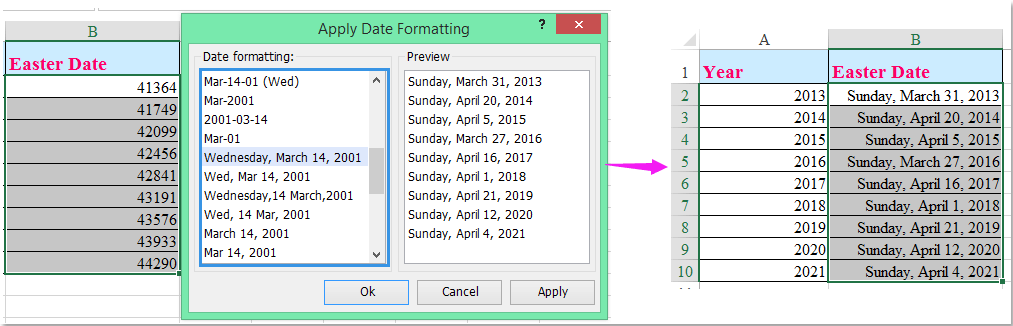####Calculate Easter date of given year with User Defined Function

The following User Defined Function also can help you to calculate the Easter date of a specific year.

1. Hold down the ALT + F11 keys, then it opens the Microsoft Visual Basic for Applications window.

2. Click Insert > Module, and paste the following code in the Module Window.

VBA code: Calculate Easter date of given year:

``````Public Function EasterDate(Yr As Integer) As Date
'Updateby Extendoffice 20160530
Dim x As Integer
x = (((255 - 11 * (Yr Mod 19)) - 21) Mod 30) + 21
EasterDate = DateSerial(Yr, 3, 1) + x + (x > 48) + 6 - ((Yr + Yr \ 4 + _
x + (x > 48) + 1) Mod 7)
End Function``````

3. Then save and close this code, go back to the worksheet and enter this formula: =easterdate(A2) into a blank cell beside your corresponding data, and then drag the fill handle down to the cells that you want to apply this formula, you will get some five-digits numbers, see screenshot: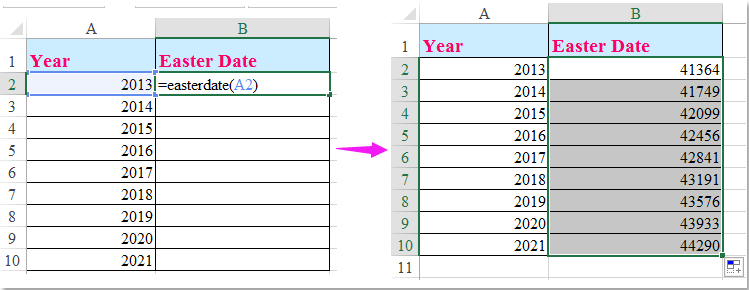5. And then you should change the numbers to date format as you need. Please view step3 of above method to format them to date.

### The Best Office Productivity Tools

#### Kutools for Excel Solves Most of Your Problems, and Increases Your Productivity by 80%

• Reuse: Quickly insert complex formulas, charts and anything that you have used before; Encrypt Cells with password; Create Mailing List and send emails...
• Super Formula Bar (easily edit multiple lines of text and formula); Reading Layout (easily read and edit large numbers of cells); Paste to Filtered Range...
• Merge Cells/Rows/Columns without losing Data; Split Cells Content; Combine Duplicate Rows/Columns... Prevent Duplicate Cells; Compare Ranges...
• Select Duplicate or Unique Rows; Select Blank Rows (all cells are empty); Super Find and Fuzzy Find in Many Workbooks; Random Select...
• Exact Copy Multiple Cells without changing formula reference; Auto Create References to Multiple Sheets; Insert Bullets, Check Boxes and more...
• Extract Text, Add Text, Remove by Position, Remove Space; Create and Print Paging Subtotals; Convert Between Cells Content and Comments...
• Super Filter (save and apply filter schemes to other sheets); Advanced Sort by month/week/day, frequency and more; Special Filter by bold, italic...
• Combine Workbooks and WorkSheets; Merge Tables based on key columns; Split Data into Multiple Sheets; Batch Convert xls, xlsx and PDF...
• More than 300 powerful features. Supports Office/Excel 2007-2019 and 365. Supports all languages. Easy deploying in your enterprise or organization. Full features 30-day free trial. 60-day money back guarantee.#### Office Tab Brings Tabbed interface to Office, and Make Your Work Much Easier

• Enable tabbed editing and reading in Word, Excel, PowerPoint, Publisher, Access, Visio and Project.
• Open and create multiple documents in new tabs of the same window, rather than in new windows.
• Increases your productivity by 50%, and reduces hundreds of mouse clicks for you every day!No ratings yet. Be the first to rate!
This comment was minimized by the moderator on the site
I translated your formula for Dutch Excel like this (year in A81):
=AFRONDEN.BENEDEN(DATUM(A81;5;DAG(MINUUT(A81/38)/2+56));7)-34

The formula gives 2079-04-16 for Easter 2079. This should be 2079-04-23. The rest of the 21st century looks ok!
This comment was minimized by the moderator on the site
Yes I've had some problems with variations on that formula. Check 2011, 2038, 2052, and 2079, all 7 days early.
This comment was minimized by the moderator on the site
Also 2108 gives 2108-Mar-31, should be 2108-Apr-1.
This comment was minimized by the moderator on the site
What about orthodox easter? Is there a code for that as well?
This comment was minimized by the moderator on the site
Is there a similar Excel Formula to figure out the Chinese New Year since it's also a Lunar Calendar Holiday?
This comment was minimized by the moderator on the site
It works on google sheets. It is very long but works without error for the problematic years too (what you mentioned here). You can copy to a cell and in K1 cell the year is expected, like 2022
=DATE(K1;IF((IFS(AND(MOD(2*MOD(K1;4)+4*MOD(K1;7)+6*MOD(19*MOD(K1;19)+24;30)+5;7)=6;MOD(19*MOD(K1;19)+24;30)=29);50;AND(MOD(2*MOD(K1;4)+4*MOD(K1;7)+6*MOD(19*MOD(K1;19)+24;30)+5;7)=6;MOD(19*MOD(K1;19)+24;30)=28;MOD(K1;19)>10);50;TRUE;22+MOD(19*MOD(K1;19)+24;30)+MOD(2*MOD(K1;4)+4*MOD(K1;7)+6*MOD(19*MOD(K1;19)+24;30)+5;7)))<=31;3;4);IF((IFS(AND(MOD(2*MOD(K1;4)+4*MOD(K1;7)+6*MOD(19*MOD(K1;19)+24;30)+5;7)=6;MOD(19*MOD(K1;19)+24;30)=29);50;AND(MOD(2*MOD(K1;4)+4*MOD(K1;7)+6*MOD(19*MOD(K1;19)+24;30)+5;7)=6;MOD(19*MOD(K1;19)+24;30)=28;MOD(K1;19)>10);50;TRUE;22+MOD(19*MOD(K1;19)+24;30)+MOD(2*MOD(K1;4)+4*MOD(K1;7)+6*MOD(19*MOD(K1;19)+24;30)+5;7)))<=31;IFS(AND(MOD(2*MOD(K1;4)+4*MOD(K1;7)+6*MOD(19*MOD(K1;19)+24;30)+5;7)=6;MOD(19*MOD(K1;19)+24;30)=29);50;AND(MOD(2*MOD(K1;4)+4*MOD(K1;7)+6*MOD(19*MOD(K1;19)+24;30)+5;7)=6;MOD(19*MOD(K1;19)+24;30)=28;MOD(K1;19)>10);49;TRUE;22+MOD(19*MOD(K1;19)+24;30)+MOD(2*MOD(K1;4)+4*MOD(K1;7)+6*MOD(19*MOD(K1;19)+24;30)+5;7));(IFS(AND(MOD(2*MOD(K1;4)+4*MOD(K1;7)+6*MOD(19*MOD(K1;19)+24;30)+5;7)=6;MOD(19*MOD(K1;19)+24;30)=29);50;AND(MOD(2*MOD(K1;4)+4*MOD(K1;7)+6*MOD(19*MOD(K1;19)+24;30)+5;7)=6;MOD(19*MOD(K1;19)+24;30)=28;MOD(K1;19)>10);49;TRUE;22+MOD(19*MOD(K1;19)+24;30)+MOD(2*MOD(K1;4)+4*MOD(K1;7)+6*MOD(19*MOD(K1;19)+24;30)+5;7)))-31))

This comment was minimized by the moderator on the site
Hello Tami,Thanks for your share. This formula does work without error on google sheets. Thanks!Sincerely,Mandy
This comment was minimized by the moderator on the site
It works on google sheets. It is very long but works without error for the problematic years too (what you mentioned here). You can copy to a cell and in K1 cell the year is expected, like 2022

=DATE(K1;IF((IFS(AND(MOD(2*MOD(K1;4)+4*MOD(K1;7)+6*MOD(19*MOD(K1;19)+24;30)+5;7)=6;MOD(19*MOD(K1;19)+24;30)=29);50;AND(MOD(2*MOD(K1;4)+4*MOD(K1;7)+6*MOD(19*MOD(K1;19)+24;30)+5;7)=6;MOD(19*MOD(K1;19)+24;30)=28;MOD(K1;19)>10);50;TRUE;22+MOD(19*MOD(K1;19)+24;30)+MOD(2*MOD(K1;4)+4*MOD(K1;7)+6*MOD(19*MOD(K1;19)+24;30)+5;7)))<=31;3;4);IF((IFS(AND(MOD(2*MOD(K1;4)+4*MOD(K1;7)+6*MOD(19*MOD(K1;19)+24;30)+5;7)=6;MOD(19*MOD(K1;19)+24;30)=29);50;AND(MOD(2*MOD(K1;4)+4*MOD(K1;7)+6*MOD(19*MOD(K1;19)+24;30)+5;7)=6;MOD(19*MOD(K1;19)+24;30)=28;MOD(K1;19)>10);50;TRUE;22+MOD(19*MOD(K1;19)+24;30)+MOD(2*MOD(K1;4)+4*MOD(K1;7)+6*MOD(19*MOD(K1;19)+24;30)+5;7)))<=31;IFS(AND(MOD(2*MOD(K1;4)+4*MOD(K1;7)+6*MOD(19*MOD(K1;19)+24;30)+5;7)=6;MOD(19*MOD(K1;19)+24;30)=29);50;AND(MOD(2*MOD(K1;4)+4*MOD(K1;7)+6*MOD(19*MOD(K1;19)+24;30)+5;7)=6;MOD(19*MOD(K1;19)+24;30)=28;MOD(K1;19)>10);49;TRUE;22+MOD(19*MOD(K1;19)+24;30)+MOD(2*MOD(K1;4)+4*MOD(K1;7)+6*MOD(19*MOD(K1;19)+24;30)+5;7));(IFS(AND(MOD(2*MOD(K1;4)+4*MOD(K1;7)+6*MOD(19*MOD(K1;19)+24;30)+5;7)=6;MOD(19*MOD(K1;19)+24;30)=29);50;AND(MOD(2*MOD(K1;4)+4*MOD(K1;7)+6*MOD(19*MOD(K1;19)+24;30)+5;7)=6;MOD(19*MOD(K1;19)+24;30)=28;MOD(K1;19)>10);49;TRUE;22+MOD(19*MOD(K1;19)+24;30)+MOD(2*MOD(K1;4)+4*MOD(K1;7)+6*MOD(19*MOD(K1;19)+24;30)+5;7)))-31))
This comment was minimized by the moderator on the site
Hello hztamas,
Thanks for your share. This formula does work without error on google sheets. Thanks!
Sincerely,
Mandy
This comment was minimized by the moderator on the site
Excel verison, more simple, you only have to change L3 at the beginning to the cell where you will put the year (like 2022) in.
=LET(Y;L3;A;MOD(Y;19);B;MOD(Y;4);C;MOD(Y;7);D;MOD(19*A+24;30);E;MOD(2*B+4*C+6*D+5;7);H;IFS(AND(E=6;D=29);50;AND(E=6;D=28;A>10);49;TRUE;22+D+E);IF(H<=31;DATE(Y;3;H);DATE(Y;4;H-31)))
This comment was minimized by the moderator on the site
Hello hztamas, Thank you a lot for your share. I changed the ";" to "," in your formula so it can work in Excel. I have data (like 2013) in cell A1.=LET(Y,A1,A,MOD(Y,19),B,MOD(Y,4),C,MOD(Y,7),D,MOD(19*A+24,30),E,MOD(2*B+4*C+6*D+5,7),H,IFS(AND(E=6,D=29),50,AND(E=6,D=28,A>10),49,TRUE,22+D+E),IF(H<=31,DATE(Y,3,H),DATE(Y,4,H-31)))This formula works, but I think it is not so much simpler than the formula in the article. Anyway, I think they are both great. Thanks again!Sincerely,Mandy
There are no comments posted here yet